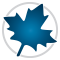Student Packages - Maple Features - Maplesoft# Student Packages

Students using a system like Maple often have different needs than non-students. They don’t just need a final answer. They are still learning the mathematical concepts behind the problems they are trying to solve, and need an environment that allows them to explore the concepts and break problems down into smaller steps instead of jumping immediately to the solution.

In addition to Clickable Math features like Drag-to-Solve, context-sensitive menus, task templates, and Smart Popups, which allow students to solve problems step-by-step without commands, Maple also features a set of student packages.   Student packages offer focused learning environments in which students can explore and reinforce fundamental concepts in the same way their instructor does in class.

For example, Maple includes step-by-step tutors that allow students to practice integration, differentiation, finding limits, and more. The Integration Tutor, shown below, lets a student compute an integral by selecting which rule to apply at each step. Maple will also offer hints or show the next step, if asked.  The tutor doesn't only demonstrate how to obtain the result, but is truly designed for practicing and learning.

Another advantage of the Maple student package concept is that computations are performed using assumptions that are appropriate for the student’s level. This avoids situations where a computation returns results that the student is unable to make sense of as the required background will be covered later in the curriculum. For example, students in a first year linear algebra course typically do not worry about complex numbers. Calculating the Euclidean norm for a vector using Maple’s Student Linear Algebra package returns the expected result:However, taking into account that symbolic quantities like a, b and c may represent complex numbers, Maple will give a more general result outside of the context of the Student package:Specialized student packages are available for calculus, precalculus, linear algebra, statistics, vector calculus, multivariate calculus, numerical analysis, and more.

Prêt(e) pour l'étape suivante ?

*Ce programme d'évaluation n'est proposé actuellement ni aux étudiants ni aux particuliers.

Achetez: